# SAS : Combining and Appending Datasets

This tutorial explains how to combine / append two data sets in SAS. In SAS, there are various method to append data sets. It can be done with data step method, PROC SQL as well as procedure called PROC APPEND to accomplish it. It is one of the most frequently data manipulation task in analytics work. For example, you have multiple human records files from various departments of your company and you are asked to join them so that there would be a single file containing information of all the departments.

## 1. Concatenate two data sets vertically / Appending Data Sets

Let's create two data sets - Data Set I and Data Set II

Data Dataset1;
Input Name \$ Score;
cards;
David 30
Ram 25
Sam 74
Sandy 36
;
run;

Data Dataset2;
Input Name \$ Score;
cards;
Ken 36
Obama 74
Raj 30
Shyam 25
;
run;

- Append / Concatenate two data sets
Data Stack;
Set Dataset1 Dataset2;
Run;

Output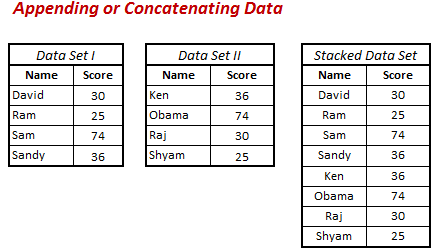Append or Join Data
Note : The stacked data set is not sorted because we have not used BY statement.

## 2. Interleaving SAS Data Sets (Sorted Stacked Data Set)

Interleaving combines individual sorted SAS data sets into one sorted data set. You interleave data sets using a SET statement and a BY statement in a DATA step.

Make sure data sets are sorted before appending datasets. Datasets can be sorted with PROC SORT.
proc sort data = dataset1;
by name;
run;
proc sort data = dataset2;
by name;
run;
Data Stack1;
Set Dataset1 Dataset2;
By Name;
Run;

OutputAppending and Sorting Together

## 3. PROC SQL for concatenating two data sets

OUTER UNION CORR keyword is used in PROC SQL to concatenate two data sets. The CORR tells SAS to append data sets by name (not by column position).

PROC SQL;
CREATE TABLE stackk AS
SELECT *
FROM Dataset1
OUTER UNION CORR
SELECT *
FROM Dataset2
ORDER BY Name;
QUIT;
The output of PROC SQL is same as the output of previous example.

## 4. PROC APPEND to concatenate data sets

In PROC APPEND, the data set specified in BASE= option refers to a data set in which other data set would be added or appended. In log, it writes 'Appending Dataset2 to Dataset1' for our example. After running this code, the dataset1 contains 8 records ( 4 from the original 'dataset1' file and 4 from the dataset2)

proc append base=dataset1 data=dataset2;
run;

If you want to append data and store it to another dataset, you can run PROC APPEND twice to do it. In the first PROC APPEND, it would create a base table ALLDATA (as specfied in the code below).  If the dataset ALLDATA does not already exist, it would be automatically created by SAS.

proc append base=alldata data=dataset1;
run;
proc append base=alldata data=dataset2;
run;
Is PROC APPEND faster?

PROC APPEND is faster than SET statement or PROC SQL UNION because it only reads in the data set being appended (i.e. the dataset identified by the syntax ‘DATA=’).

Application of PROC APPEND

PROC APPEND is most useful when you use it in a macro. For example, you create a macro in which there is a loop for calculation of some metrics of multiple variables. In each iteration, it returns a data set which needs to be appended with the output of subsequent iterations so that once loop completes all the iterations, we would have complete data set.

Important Point

Suppose you have two datasets having same variable names but the length of the common variable is different, It would throw a warning and it would not append datasets. To workaround this issue, we can use FORCE option to append the data sets.

proc append base=dataset1 data=dataset2 force;
run;

## 5. Usage of Multiple Set Statement

Instead of specifying multiple data sets in a single SET statement, we can also multiple SET statements but the result /output would be totally different. See the output shown below -

Data Stack3;
Set Dataset1;
Set Dataset2;
Run;
Warning : It overwrites data.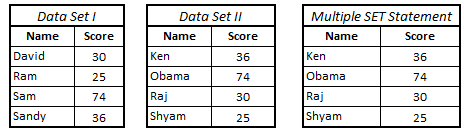Multiple SET Statements

The question arises " why do we use multiple set statement if it overwrites data". The next topic describes the application of multiple set statement.
Application of Multiple Set Statement

It combines data sets by adding column from the other dataset. Unlike INNER/LEFT Joins, it does not require any primary or unique key to join two datasets.

Lets create a new data set - Data Set 3
Data Dataset3;
Input Section \$;
cards;
A
B
C
D
;
run;
Data Stack3;
Set Dataset1;
Set Dataset3;
Run;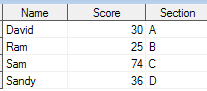Multiple SET Statemet

Append Data : Many to Many Relationship

Multiple SET statement can also produce cartesian product of two tables. It can be done with POINT= option.

Data Stack2;
set Dataset1;
do i=1 to num;
set Dataset3 nobs=num point=i;
if i=i then output;
end;
run;Many to Many Relationships
Related Posts
ShareDeepanshu founded ListenData with a simple objective - Make analytics easy to understand and follow. He has over 10 years of experience in data science. During his tenure, he worked with global clients in various domains like Banking, Insurance, Private Equity, Telecom and HR.

4 Responses to "SAS : Combining and Appending Datasets"
1.Hi,
what is the effect if we don't sort the data sets before appending?

1.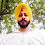If you are using BY statement in merging but dont sort the data then the code will error out.

2.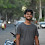This comment has been removed by the author.

2.What does i=i do in the last code? Won't it always result in TRUE?

Next → ← Prev